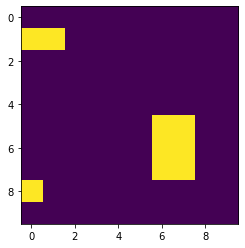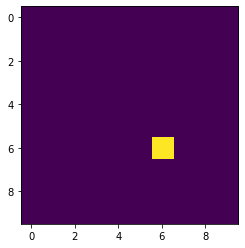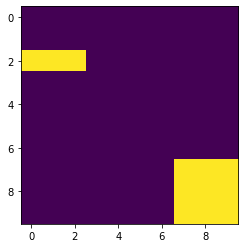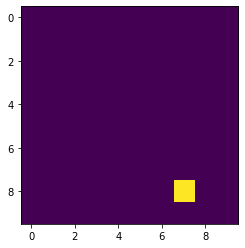# Mahotas – Hit & Miss transform

In this article we will see how we can perform hit and miss transform in mahotas. In mathematical morphology, hit-or-miss transform is an operation that detects a given configuration in a binary image, using the morphological erosion operator and a pair of disjoint structuring elements.

In order to do this we will use ` mahotas.hitmiss` method

Syntax : mahotas.hitmiss(img, template)

Argument : It takes two numpy ndarray as argument

Return : It returns ndarray

Below is the implementation

 `# importing required libraries ` `import` `mahotas as mh ` `import` `numpy as np ` `from` `pylab ``import` `imshow, show ` `   `  `# creating region ` `# numpy.ndarray ` `regions ``=` `np.zeros((``10``, ``10``), ``bool``) ` `   `  `# setting 1 value to the region ` `regions[``1``, :``2``] ``=` `1` `regions[``5``:``8``, ``6``: ``8``] ``=` `1` `regions[``8``, ``0``] ``=` `1` `     `  `# showing the image with interpolation = 'nearest' ` `print``(``"Image"``) ` `imshow(regions, interpolation ``=``'nearest'``) ` `show() ` `  `  `# template for hit miss ` `template ``=` `np.array([ ` `            ``[``0``, ``1``, ``1``], ` `            ``[``0``, ``1``, ``1``], ` `            ``[``0``, ``1``, ``1``]]) ` `  `  `# hit miss transform ` `img ``=` `mahotas.hitmiss(regions, template) ` ` `  `# showing image ` `print``(``"Image after hit miss transform"``) ` `imshow(img) ` `show() `

Output :

`Image``Image after hit miss transform`Another example

 `# importing required libraries ` `import` `mahotas as mh ` `import` `numpy as np ` `from` `pylab ``import` `imshow, show ` `   `  `# creating region ` `# numpy.ndarray ` `regions ``=` `np.zeros((``10``, ``10``), ``bool``) ` `   `  `# setting 1 value to the region ` `regions[``2``:``3``, :``3``] ``=` `1` `regions[``7``:, ``7``:] ``=` `1` `     `  `# showing the image with interpolation = 'nearest' ` `print``(``"Image"``) ` `imshow(regions, interpolation ``=``'nearest'``) ` `show() ` `  `  `# template for hit miss ` `template ``=` `np.array([ ` `            ``[``0``, ``1``, ``1``], ` `            ``[``0``, ``1``, ``1``], ` `            ``[``0``, ``1``, ``1``]]) ` `  `  `# hit miss transform ` `img ``=` `mahotas.hitmiss(regions, template) ` ` `  `# showing image ` `print``(``"Image after hit miss transform"``) ` `imshow(img) ` `show() `

Output :

`Image``Image after hit miss transform`Attention geek! Strengthen your foundations with the Python Programming Foundation Course and learn the basics.

To begin with, your interview preparations Enhance your Data Structures concepts with the Python DS Course.

My Personal Notes arrow_drop_upCheck out this Author's contributed articles.

If you like GeeksforGeeks and would like to contribute, you can also write an article using contribute.geeksforgeeks.org or mail your article to contribute@geeksforgeeks.org. See your article appearing on the GeeksforGeeks main page and help other Geeks.

Please Improve this article if you find anything incorrect by clicking on the "Improve Article" button below.

Article Tags :

1

Please write to us at contribute@geeksforgeeks.org to report any issue with the above content.